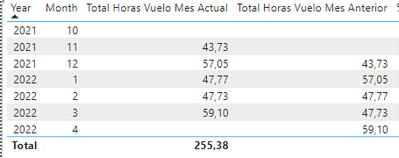cancel
Showing results for
Did you mean:Frequent Visitor

## Current Month vs Previous Month and values when changing the year

Hello,

I am trying to compare 2 columns:

- Current month values

vs.

- Previous Month Values

To do that, I´ve developed 2 Measures:

- Total of Current Flight Hours

``````Total Horas Vuelo Mes Actual =
CALCULATE(
(SUM('PROGRAMACIÓN REAL ENERO 2022'[Duracion del vuelo (sg)])/3600))``````

and

- Total of Previous Month Flight Hours

``````Total Horas Vuelo Mes Anterior =
CALCULATE(Medidas[Total Horas Vuelo Mes Actual],
FILTER(ALL(dDate),
dDate[Año]=SELECTEDVALUE(dDate[Año]) &&
dDate[MesNro]=SELECTEDVALUE(dDate[MesNro])-1)
)``````

I also have the porcentage (%) between Flight hours of current month and previous month:

``%Dif. Horas de Vuelo Mes Actual = (([Total Horas Vuelo Mes Actual]-[Total Horas Vuelo Mes Anterior])/([Total Horas Vuelo Mes Anterior]))``

However when I´m visualizing those three columns, when changing the year (for example, between Dec 2021 and Jan 2022), previous month is BLANK (see the picture attached)How can I manage it to get the value from December???

1 ACCEPTED SOLUTIONSuper User

You need to add another column [Year Month Sequential Number]

=
RANKX ( TableName, [Year_Month_ Value],, ASC, Dense )

5 REPLIES 5Super User

when you have the month January, the month number is 1 therefore, 1 - 1 is 0 which is a month that do not exist. Therefore, the result is blank. You need to use a Year Month sequential number column in your date table which can simply be a dense rank (ascending) of the year month number. And the year month number can be just YEAR ([Date] ) *100 + MONTH ( [Date] ). Please let me know if you need any help on this.Frequent Visitor

Thank you Tamerj1!

I´ve created a new column (Year_Month_Value) in the calendar table (dDate)

``Year_Month_Value = dDate[Year]*100+dDate[MonthNumber]``The current Flight Hours is:

``````Total Horas Vuelo Mes Actual =
CALCULATE(
(SUM('PROGRAMACIÓN REAL ENERO 2022'[Duracion del vuelo (sg)])/3600))``````

So for column "Previous Flight Hours" I´ve also wrote:

``````Total Horas Vuelo Mes Anterior =
CALCULATE(Medidas[Total Horas Vuelo Mes Actual],
FILTER(ALL(dDate),
dDate[Year_Month_Value]=SELECTEDVALUE(dDate[Year_Month_Value])-1)
)``````

However, on January is not appearing the previous month as the picture shows:Super User

You need to add another column [Year Month Sequential Number]

=
RANKX ( TableName, [Year_Month_ Value],, ASC, Dense )Frequent Visitor

Great! That works!

I´ll start to use RANKX!Super User

You should use the time-intel functions to do such calculations. Doing them by hand (like you do) will invariably lead to problems (and they just have). Please start here: Time Intelligence in Power BI Desktop - SQLBI

Your issue stems from the fact that you're trying to subtract 1 from 1 (the number for January), which is 0, but it should be 12 (to go to December last year). But it's messy and error-prone to do such calculations by hand, as I said. Please use time-intel. That's what it's for.